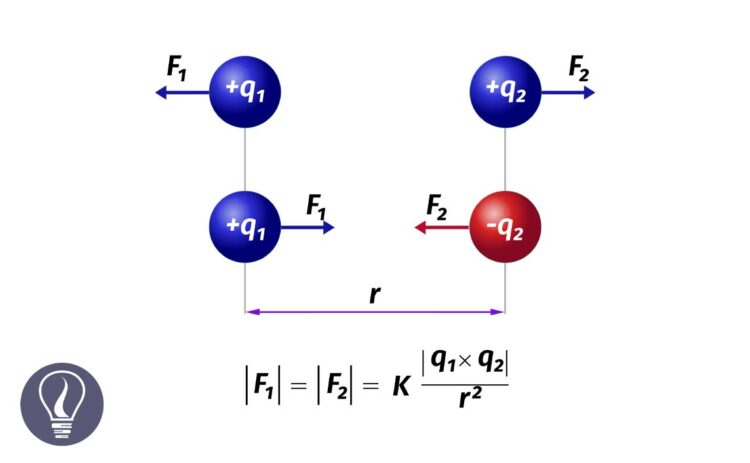More results...

Generic selectors
Exact matches only
Search in title
Search in content
Post Type Selectors

# Coulomb's LawB.Sc (Hons, USJ) (Polymer Science and Technology, Chemistry, Physics)
Categories
Last Updated On : September 22, 2023
Published Date : September 6, 2023

## The Coulomb's Law (Coulomb's inverse-square law)

The mutual electric force acting between two pointed stationary electric charges is proportional to the magnitude of the respective charges and inversely proportional to the square of the displacement between the two charges.

A repulsive force acts between two charges, if the two charges are like charges. An attraction force acts if the two charges are unlike charges. This force is called the Coulomb force.

If there are two charges as q1 and q2 at a displacement of r, the Coulomb's law can be expressed mathematically as follows,

Where,

• F = Coulomb force
• q1, q2 = charge of the pointed charges (C)
• r = displacement between two charges
• k = Coulomb constant : 8.99 × 109 Nm2C-2

In a vacuum, the value of k is 8.99 × 109 N m2 C-2. Coulomb's constant is calculated as follows. Where K is the Coulomb constant and ε is the permittivity of the medium.

## Permittivity

Permittivity is the ability of a material to store electric energy under an external electric field. Simply it describes how much a medium or a material allows electric field lines to pass through them. If the permittivity is low, that means the medium does not allow many electric field lines to pass through it and the medium can store low electric energy.

A vacuum has the lowest possible permittivity.

The permittivity of a vacuum = 8.85 × 10-12 F m-1

Metals have an infinite permittivity. That means metals allow infinite electric field lines to pass through them.

Mathematically,

The permeability of a medium can affect the force existing between two point charges in the medium. The force is inversely proportional to the permittivity of the medium.

## Relative permittivity / Dielectric constant

Generally, the permittivity is expressed relative to the permittivity of a vacuum. Relative permittivity or the dielectric constant of a medium is the ratio between the permittivity of a medium and the permittivity of a vacuum. The permittivity of a material is always higher than 1.

Mathematically,

Where,

• εr = Relative permittivity / Dielectric constant
• ε = Permittivity of the medium
• ε0 = Permittivity of a vacuum

The coulomb force for two pointed charges can be expressed in terms of permittivity or relative permittivity.

In terms of relative permittivity or dielectric constant,

## Solving questions/problems related to Coulomb's Law

Question 01

Find the repulsive force acts on two positively charged pointed charges each having +2 μC, when the two charges are placed in a vacuum at 2 cm displacement.

Solution

Question 02

An electron is released from rest at a distance of 1 cm from a point stationary charge which has a charge of +2 μC. Find the percentage increase in force acts of the electron when it is 1mm away from the stationary charge.

Solution

For the entire motion, the permittivity of the medium (ε), charges of the electron and the charge of the pointed charge (q1, q2) do not change. Therefore, for the entire motion q1q2/ 4πε is a constant. If we take this constant as k, we can write the coulomb's formula as follows,

• When the electron is 1 cm away from the pointed charge,

• When the electron is 1mm away from the pointed charge,## References and Attributes

### Figures:

The cover image was designed using an image by Dna-Dennis, licensed under CC BY 3.0, via Wikimedia Commons